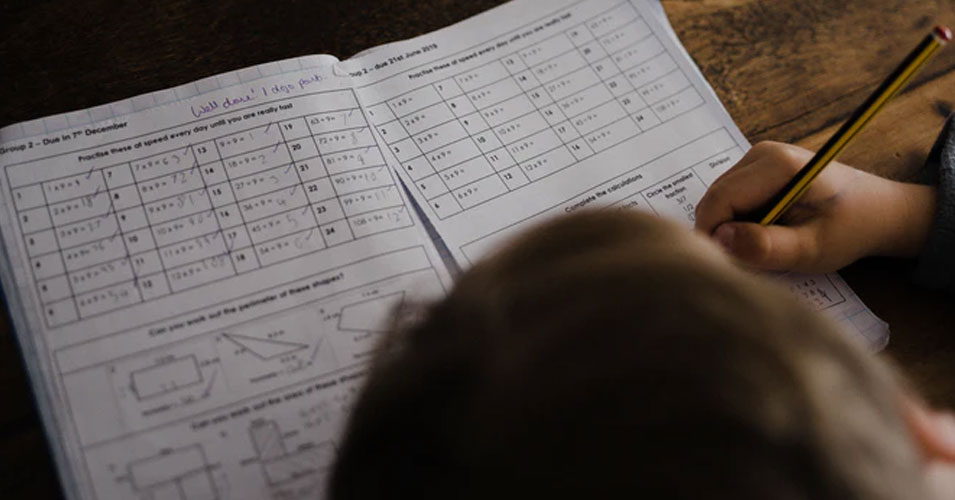• Save

# Geometry Aptitude Questions for Competitive ExamsDears Friends, As you know, Geometry questions are often asked in competitive exams like SSC, IAS, RAS, UPSC, RRB, BANK, which is the main part of the Aptitude section. It broadly tests an examinee on concepts. Geometry is that branch of mathematics in which the studied of points, lines, curves, planes, etc. If you expect to score almost all the marks in Geometry questions, then you must first understand the complexity of these questions.

Here today, in this blog, we are providing important Geometry Aptitude questions for better preparation of students in competitive exams, which can strengthen your preparation in Competition Paper. With the help of the questions given here, you can practice well by solving geometry questions.

## Important Geometry Aptitude Questions

Q :

An octahedron has 12 edges, How many vertices does it have,

(A) 10

(B) 6

(C) 8

(D) 18

Q :

If the circumradius of an equilateral triangle ABC be 8 cm, then the height of the triangle is

(A) 8 cm

(B) 12 cm

(C) 16 cm

(D) 6 cm

Q :

The distance between the center of two equal circles each of radius 3 cm, is 10 cm. The length of a transverse common tangent is

(A) 4 cm

(B) 10 cm

(C) 8 cm

(D) 6 cm

Q :

The length of diagonal BD of a parallelogram ABCD is 36 cm. P and Q are the centroids of triangle ABC and triangle ADC respectively. What is the length (in cm) of PQ?

(A) 26

(B) 39

(C) 13

(D) 52

Q :

The radius of the circumcircle of a right-angled triangle is 15 cm and the radius of its in circle is 6 cm. Find the sides of the triangle.

(A) 30 , 24 , 25

(B) 24 , 36 , 20

(C) 30 , 40 , 41

(D) 18 , 24 , 30

Q :

A unique circle can always be drawn through x number of given non-collinear points, then X must be

(A) 4

(B) 1

(C) 2

(D) 3

Q :

In triangle ABC, AD, BE and CF are the medians intersecting at point G and area of triangle ABC is 156 cm2. what is the area (in cm2) of triangle FGE?

(A) 26

(B) 39

(C) 13

(D) 52

Q :

The maximum number of common tangents drawn to two circles when both the circles touch each other externally is

(A) 3

(B) 0

(C) 1

(D) 2

Q :

The length of the common chord of two intersecting circles is 24 cm. If the diameter of the circles are 30 cm and 26 cm, then the distance between the center (in cm) is

(A) 15

(B) 16

(C) 13

(D) 14

Q :

An arc of 30 ° in one circle is double an arc in a second circle, the radius of which is three times the radius of the first. Then the angles subtended by the arc of the second circle at its center is

(A) 5°

(B) 6°

(C) 3°

(D) 4°